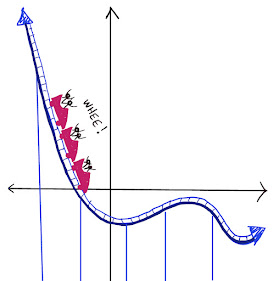## Tuesday, March 15, 2022

### Lattice Polygons, Part 2

Lattice polygons are polygons are formed by connecting dots on square dot paper.  (On a coordinate graph, the coordinates of the vertices are integers.).

The lattice polygon below is a rectangle whose dimensions are 2 units by 4 units.  Its area is 8 square units.

(1)  Can you make a small change to the rectangle so that the area of the new polygon is 6 square units? There are lots of ways to do this.

(2)  How many different kinds of polygons can you make on a geoboard with an area of exactly 6 square units?  Can you make a triangle?  a quadrilateral?  a 5-sided figure?  6-sided?  7-sided?  8-sided?

It is convenient to use this online geoboard:  Geoboard

For the solution, click below:

## Thursday, March 10, 2022

### Same Sum Circles, Part 2

The goal of this puzzle is to place the numbers 1 through 7 in the circles so that you get the same sum in each circle.

Notice that the placement below is ALMOST a solution but not quite.  One circle has a sum of 17 and the other two have a sum of 19.  You can fix it by moving only one bean.  Which one?  Where would you move it?

Once you figure that out, see how many different sums you can make.  You can make quite a lot!

Click "read more" for the solution:

### PLAYFUL MATH BLOG CARNIVAL #163

BLOG CARNIVAL #163....LET'S GO! Fun fact: The number 163 is prime, which we can prove simply by showing that it is not divisible by 2, 3...